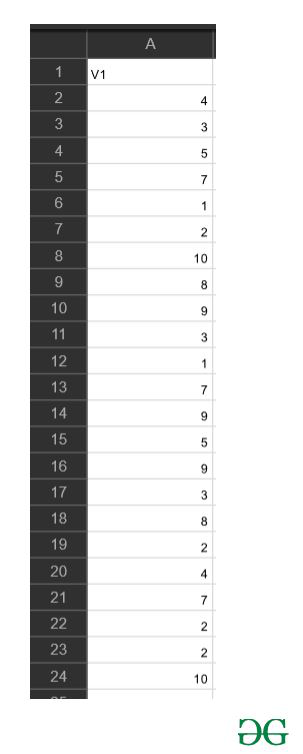How to calculate mean of a CSV file in R?

• Last Updated : 26 Mar, 2021

Mean or average is a method to study central tendency of any given numeric data. It can be found using the formula.In this article, we will be discussing two different ways to calculate the mean of a CSV file in R.

Data in use:Method 1: Using mean function

In this method to calculate the mean of the column of a CSV file we simply use the mean() function with the column name as its parameter and this function will be returning the mean of the provided column of the CSV file.

Syntax:

mean(name of the column)

Approach

• Select column
• Pass the column to mean function
• Display result

Example:

R

Output:

 5.266667

Method 2: Using  nrow() and sum()

In this method we will be using the sum and the nrow functions separately to calculate the total number of entity in the whole csv file and there respected sum and then divide the total sum by the number of rows to get the mean.

• sum() function:-Returns the sum of the respected parameter

Syntax:

sum(column_name)

• nrow() function:-Returns the number of entity in the CSV file

Syntax:

nrow(name of the file)

Approach

• Import file
• Find sum
• Find frequency
• Divide sum by frequency
• Store this value as mean
• Display result

Example: